• python绘制三维
2020-08-27 21:31:10

## python绘制三维平面图

用python绘制3维图片，示例为关于公式
f ( x ， y ) = ( x 2 + y − 11 ) 2 + ( x + y 2 − 7 ) 2 \\f({x，y} )= (x^2+y-11)^2+(x+y^2-7)^2

如果报错：Unknown projection ‘3d’
则必须要加上：from mpl_toolkits.mplot3d import Axes3D

import matplotlib.pyplot as plt
import numpy as np
from mpl_toolkits.mplot3d import Axes3D
%matplotlib inline
# f(x,y) = (x^2+y-11)^2+(x+y^2-7)^2
def himmelblau(x):
return (x**2+x-11)**2+(x+x**2-7)**2
x = np.arange(-6,6,0.1)
y = np.arange(-6,6,0.1)
X,Y = np.meshgrid(x,y)
print('x,y maps',X.shape,Y.shape)
Z = himmelblau([X,Y])

fig = plt.figure('himmelblau')
ax = fig.gca(projection='3d')
ax.plot_surface(X,Y,Z)
ax.view_init(60,-30)
ax.set_xlabel('x')
ax.set_ylabel('y')
plt.show()更多相关内容
• 1. python三维图表绘制方法简介python三维图表绘制算是二维图表的一个进阶版本，本质上和二维图表的绘制并无差别，唯一的区别在于使用的库略有差异。相较于二维图表使用的pyplot库，三维图表绘制使用的是Axes3D...

1. python三维图表绘制方法简介

python三维图表的绘制算是二维图表的一个进阶版本，本质上和二维图表的绘制并无差别，唯一的区别在于使用的库略有差异。

相较于二维图表使用的pyplot库，三维图表的绘制使用的是Axes3D库。

库引入语句为：

from matplotlib import pyplot as plt

from mpl_toolkits.mplot3d import Axes3D

上下的操作就和二维图表绘制大差不差了，首先定义三维画布，然后向里面绘制三维图表，最后打印出结果即可。

下面，我们通过一些实例来进行说明。

2. 实例说明

1. 三维曲线图绘制

首先，我们来看一下三维曲线图的绘制。

三维曲线图的绘制和二维曲线图的绘制方法极其相似，只是我们需要做以下两点修改：

将画布修改为三维坐标系；

传参时同时传入x、y、z三个维度的坐标信号。

另外，plot函数需要修改三维曲线绘制的Axes3D.plot函数。

给出代码样例如下：

import numpy

import matplotlib.pyplot as plt

from mpl_toolkits.mplot3d import Axes3D

theta = numpy.linspace(0, 3.14*4, 200)

r = numpy.linspace(0, 1, 200)

x = r * numpy.cos(theta)

y = r * numpy.sin(theta)

z = numpy.linspace(0, 2, 200)

fig = plt.figure(figsize=(12, 7))

ax1 = plt.axes(projection='3d')

ax1.plot(x, y, z)

plt.show()

运行即可得到一张三维曲线图。2. 三维散点图绘制

下面，我们来看一下三维空间中的散点图绘制方法。

其方法其实挺简单的，就是先绘制x、y面的网点坐标，计算相应的z轴高度，而后创建一张三维图，然后通过Axes3D.scatter函数进行散点图绘制即可。

我们给出具体的代码样例如下：

import numpy

import matplotlib.pyplot as plt

from mpl_toolkits.mplot3d import Axes3D

x = numpy.linspace(-2, 2, 10)

y = numpy.linspace(-2, 2, 10)

xx, yy = numpy.meshgrid(x, y)

z = xx ** 2 - yy ** 2

fig = plt.figure(figsize=(12, 7))

ax1 = plt.axes(projection='3d') # 创建三维坐标轴

ax1.scatter(xx, yy, z) # 绘制三维散点图

plt.show()

运行即可得到三维散点图。3. 三维曲面图绘制

三维曲面图的绘制与三维极其类似，只需要将Axes3D.scatter函数替换为Axes3D.plot_surface函数即可。

我们就不再多做解释了，直接给出代码样例如下：

import numpy

import matplotlib.pyplot as plt

from mpl_toolkits.mplot3d import Axes3D

x = numpy.linspace(-2, 2, 10)

y = numpy.linspace(-2, 2, 10)

xx, yy = numpy.meshgrid(x, y)

print(xx.shape, yy.shape)

z = xx ** 2 - yy ** 2

fig = plt.figure(figsize=(12, 7))

ax1 = plt.axes(projection='3d') # 创建三维坐标轴

ax1.plot_surface(xx, yy, z) # 绘制三维曲面图

plt.show()

运行即可得到三维曲面图。3. 参考链接

展开全文• 三维图表

Matplotlib 最开始被设计为仅支持二维的图表。到 1.0 版本发布左右，一些三维图表的工具在二维展示的基础上被创建了出来，结果就是 Matplotlib 提供了一个方便的（同时也是有限的）的可用于三维数据可视化的一套工具。三维图表可以使用载入mplot3d工具包来激活，这个包会随着 Matplotlib 自动安装：

from mpl_toolkits import mplot3d


一旦模块被导入，三维 axes 就可以像其他普通 axes 一样通过关键字参数projection='3d’来创建：

%matplotlib inline
import numpy as np
import matplotlib.pyplot as plt

fig = plt.figure()
ax = plt.axes(projection='3d')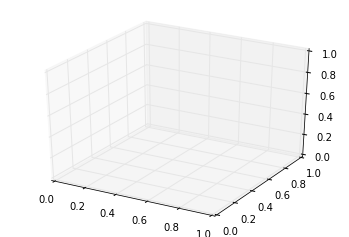三维 axes 激活后，我们可以在上面绘制不同的三维图表类型。三维图表在 notebook 中使用交互式图表展示会优于使用静态展示；回忆我们前面介绍过，你可以使用%matplotlib notebook而不是%matplotlib inline来激活交互式展示模式。

## 三维的点和线

三维图表中最基础的是使用(x, y, z)坐标定义的一根线或散点的集合。前面介绍过普通的二维图表，作为类比，使用ax.plot3D和ax.scatter3D函数可以创建三维折线和散点图。这两个函数的签名与二维的版本基本一致，你可以参考我的文章[简单折线图]和[简单散点图]来复习一下这部分的内容。下面我们绘制一个三维中的三角螺旋，在线的附近在绘制一些随机的点：

ax = plt.axes(projection='3d')

# 三维螺旋线的数据
zline = np.linspace(0, 15, 1000)
xline = np.sin(zline)
yline = np.cos(zline)
ax.plot3D(xline, yline, zline, 'gray')

# 三维散点的数据
zdata = 15 * np.random.random(100)
xdata = np.sin(zdata) + 0.1 * np.random.randn(100)
ydata = np.cos(zdata) + 0.1 * np.random.randn(100)
ax.scatter3D(xdata, ydata, zdata, c=zdata, cmap='Greens');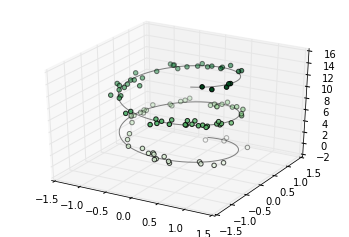注意默认情况下，图中的散点会有透明度的区别，用于体现在图中散点的深度。虽然三维效果在静态图像中难以显示，你可以使用交互式的视图来获得更佳的三维直观效果。

## 三维轮廓图

类似于我们在[密度和轮廓图]中介绍的内容，mplot3d也包含着能够创建三维浮雕图像的工具。就像二维的ax.contour图表，ax.contour3D要求输入数据的格式是二维普通网格上计算得到的 Z 轴的数据值。下面我们展示一个三维的正弦函数轮廓图：

def f(x, y):
return np.sin(np.sqrt(x ** 2 + y ** 2))

x = np.linspace(-6, 6, 30)
y = np.linspace(-6, 6, 30)

X, Y = np.meshgrid(x, y)
Z = f(X, Y)

fig = plt.figure()
ax = plt.axes(projection='3d')
ax.contour3D(X, Y, Z, 50, cmap='binary')
ax.set_xlabel('x')
ax.set_ylabel('y')
ax.set_zlabel('z');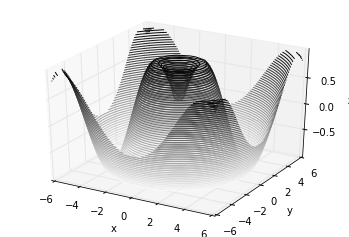有时候默认的视角角度不是最理想的，在这种情况下我们可以使用view_init函数来设置水平角和方位角。在下面的例子中，我们使用的是 60° 的水平角（即以 60° 俯视 x-y 平面）和 35° 的方位角（即将 z 轴逆时针旋转 35°）：

ax.view_init(60, 35)
fig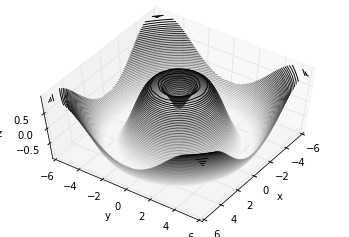同样，注意到当使用 Matplotlib 交互式展示是，这样的旋转可以通过鼠标点击和拖拽来实现。

## 框线图和表面图

使用网格数据生成的三维图表还有框线图和表面图。这两种图表将网格数据投射到特定的三维表面，能够使得结果图像非常直观和具有说服力。下面是一个框线图的例子：

fig = plt.figure()
ax = plt.axes(projection='3d')
ax.plot_wireframe(X, Y, Z, color='black')
ax.set_title('wireframe');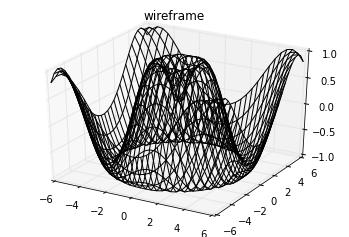表面图类似框线图，区别在于每个框线构成的多边形都使用颜色进行了填充。添加色图用于填充多边形能够让图形表面展示出来：

ax = plt.axes(projection='3d')
ax.plot_surface(X, Y, Z, rstride=1, cstride=1,
cmap='viridis', edgecolor='none')
ax.set_title('surface');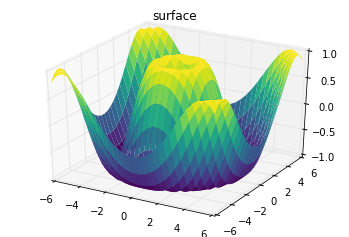注意虽然每个颜色填充的表面都是二维的，但是表面的边缘不需要是直线构成的。下面的例子使用surface3D绘制了一个部分极坐标网格，能够让我们切入到函数内部观察效果：

r = np.linspace(0, 6, 20)
theta = np.linspace(-0.9 * np.pi, 0.8 * np.pi, 40)
r, theta = np.meshgrid(r, theta)

X = r * np.sin(theta)
Y = r * np.cos(theta)
Z = f(X, Y)

ax = plt.axes(projection='3d')
ax.plot_surface(X, Y, Z, rstride=1, cstride=1,
cmap='viridis', edgecolor='none');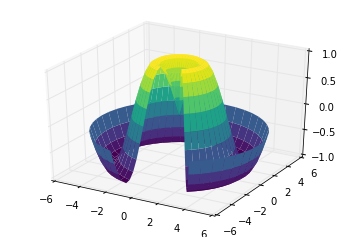## 表面三角剖分

在一些应用场合中，上面的这种均匀网格绘制的图表方式太过于局限和不方便。在这些情况下，三角剖分的图表可以派上用场。如果我们并不是使用笛卡尔坐标系或极坐标系的网格来绘制三维图表，而是使用一组随机的点来绘制三维图表呢？

theta = 2 * np.pi * np.random.random(1000)
r = 6 * np.random.random(1000)
x = np.ravel(r * np.sin(theta))
y = np.ravel(r * np.cos(theta))
z = f(x, y)


有了上面的数据之后，我们可以使用它们来绘制一张散点图表现出样本所在表面的情况：

ax = plt.axes(projection='3d')
ax.scatter(x, y, z, c=z, cmap='viridis', linewidth=0.5);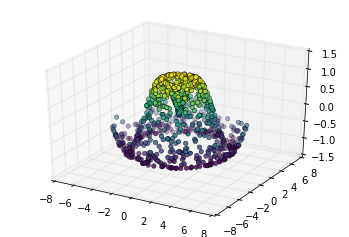上图并未形象的表示出表面情况。这种情况下我们可以使用ax.plot_trisurf函数，它能首先根据我们的数据输入找到各点内在的三角函数形式，然后绘制表面（注意的是这里的 x，y，z 是一维的数组）：

ax = plt.axes(projection='3d')
ax.plot_trisurf(x, y, z,
cmap='viridis', edgecolor='none');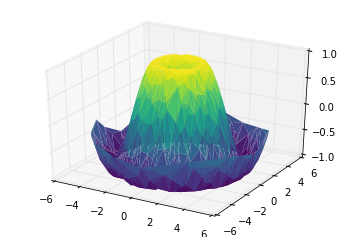上图的结果很显然没有使用网格绘制表面图那么清晰，但是对于我们并不是使用函数构建数据样本（数据样本通常来自真实世界的采样）的情况下，这能提供很大的帮助。例如我们下面会看到，能使用这种方法绘制一条三维的莫比乌斯环。

## 例子：绘制莫比乌斯环

莫比乌斯环是使用一条纸条，一端翻折后与另一端粘起来形成的环形。在拓扑学中这是非常有趣的一个形状，因为它只有一个面。我们下面使用 Matplotlib 的三维工具绘制莫比乌斯环。创建莫比乌斯环的关键在于能参数化它：莫比乌斯环是一个二维的环状结构，因此我们需要两个特定的维度。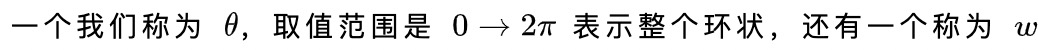取值范围是（-1,1），表示带子宽度：

theta = np.linspace(0, 2 * np.pi, 30)
w = np.linspace(-0.25, 0.25, 8)
w, theta = np.meshgrid(w, theta)


有了这两个参数之后，我们需要确定莫比乌斯环上*(x, y, z)*坐标的位置。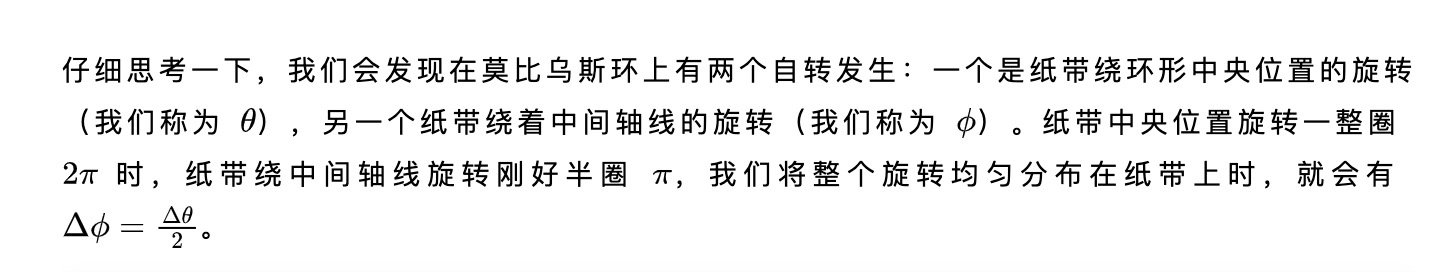phi = 0.5 * theta# r是坐标点距离环形中心的距离值
r = 1 + w * np.cos(phi)
# 利用简单的三角函数知识算得x，y，z坐标值
x = np.ravel(r * np.cos(theta))
y = np.ravel(r * np.sin(theta))
z = np.ravel(w * np.sin(phi))


最后，为了绘制对象，我们必须保证三角剖分是正确的。实现这个最好的方法是在底层的参数上面实现三角剖分，最后让 Matplotlib 将这个三角剖分投射到三维空间中形成莫比乌斯环。下面的代码最终绘制图形：

# 在底层参数的基础上进行三角剖分
from matplotlib.tri import Triangulation
tri = Triangulation(np.ravel(w), np.ravel(theta))

ax = plt.axes(projection='3d')
ax.plot_trisurf(x, y, z, triangles=tri.triangles,
cmap='viridis', linewidths=0.2);

ax.set_xlim(-1, 1); ax.set_ylim(-1, 1); ax.set_zlim(-1, 1);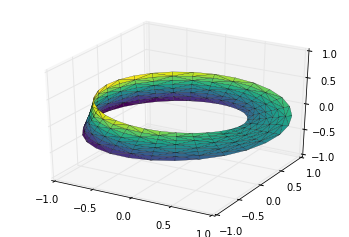展开全文数据可视化
• 1. python三维图表绘制方法简介python三维图表绘制算是二维图表的一个进阶版本，本质上和二维图表的绘制并无差别，唯一的区别在于使用的库略有差异。相较于二维图表使用的pyplot库，三维图表绘制使用的是Axes3D...

1. python三维图表绘制方法简介

python三维图表的绘制算是二维图表的一个进阶版本，本质上和二维图表的绘制并无差别，唯一的区别在于使用的库略有差异。

相较于二维图表使用的pyplot库，三维图表的绘制使用的是Axes3D库。

库引入语句为：

1

2

from import pyplot as plt

from mpl_toolkits.mplot3dimport Axes3D

上下的操作就和二维图表绘制大差不差了，首先定义三维画布，然后向里面绘制三维图表，最后打印出结果即可。

下面，我们通过一些实例来进行说明。

2. 实例说明

1. 三维曲线图绘制

首先，我们来看一下三维曲线图的绘制。

三维曲线图的绘制和二维曲线图的绘制方法极其相似，只是我们需要做以下两点修改：

将画布修改为三维坐标系；

传参时同时传入x、y、z三个维度的坐标信号。

另外，plot函数需要修改三维曲线绘制的Axes3D.plot函数。

给出代码样例如下：

1

2

3

4

5

6

7

8

9

10

11

12

13

14

import numpy

import matplotlib.pyplot as plt

from mpl_toolkits.mplot3dimport Axes3D

theta= numpy.linspace(0,3.14*4,200)

r= numpy.linspace(0,1,200)

x= r* numpy.cos(theta)

y= r* numpy.sin(theta)

z= numpy.linspace(0,2,200)

fig= plt.figure(figsize=(12,7))

ax1= plt.axes(projection='3d')

ax1.plot(x, y, z)

plt.show()

运行即可得到一张三维曲线图。2. 三维散点图绘制

下面，我们来看一下三维空间中的散点图绘制方法。

其方法其实挺简单的，就是先绘制x、y面的网点坐标，计算相应的z轴高度，而后创建一张三维图，然后通过Axes3D.scatter函数进行散点图绘制即可。

我们给出具体的代码样例如下：

1

2

3

4

5

6

7

8

9

10

11

12

13

import numpy

import matplotlib.pyplot as plt

from mpl_toolkits.mplot3dimport Axes3D

x= numpy.linspace(-2,2,10)

y= numpy.linspace(-2,2,10)

xx, yy= numpy.meshgrid(x, y)

z= xx** 2 - yy** 2

fig= plt.figure(figsize=(12,7))

ax1= plt.axes(projection='3d')# 创建三维坐标轴

ax1.scatter(xx, yy, z)# 绘制三维散点图

plt.show()

运行即可得到三维散点图。3. 三维曲面图绘制

三维曲面图的绘制与三维极其类似，只需要将Axes3D.scatter函数替换为Axes3D.plot_surface函数即可。

我们就不再多做解释了，直接给出代码样例如下：

1

2

3

4

5

6

7

8

9

10

11

12

13

14

import numpy

import matplotlib.pyplot as plt

from mpl_toolkits.mplot3dimport Axes3D

x= numpy.linspace(-2,2,10)

y= numpy.linspace(-2,2,10)

xx, yy= numpy.meshgrid(x, y)

print(xx.shape, yy.shape)

z= xx** 2 - yy** 2

fig= plt.figure(figsize=(12,7))

ax1= plt.axes(projection='3d')# 创建三维坐标轴

ax1.plot_surface(xx, yy, z)# 绘制三维曲面图

plt.show()

运行即可得到三维曲面图。3. 参考链接

到此这篇关于matplotlib 三维图表绘制方法简介的文章就介绍到这了,更多相关matplotlib 三维图表内容请搜索服务器之家以前的文章或继续浏览下面的相关文章希望大家以后多多支持服务器之家！

原文链接：https://blog.csdn.net/codename_cys/article/details/108686439

展开全文• Barplot Axes3D.bar(left, height, zs=0, zdir=‘z’, *args, **kwargs)  其他参数向下传递给bar函数，返回Patch3DCollection对象 Argument Description left 条形图水平坐标 ...from mpl_toolkits.mplot...开发语言
• 代码 ''' Author: CloudSir Date: 2021-07-28 10:...Description: python绘制3D图表 https://github.com/cloudsir ''' from mpl_toolkits.mplot3d import Axes3D import numpy as np from matplotlib import pyplot as数据可视化
• Python matplotlib 绘制3D柱形图](https://www.jianshu.com/p/b1e13b057a17)from mpl_toolkits.mplot3dimport Axes3Dimport matplotlib.pyplotas pltimport numpyas np#设置x轴取值xedges = np.array([10,20,30,40,...
• 网上搜了一下关于python绘制三维柱状图，没有找到可用的，偶然间发现一个第三方库pyecharts，不仅可以绘制三维图，还能动态展示，生成的是html文件。 中文文档地址：http://pyecharts.herokuapp.com/；github项目...数据可视化 数据挖掘
• Python绘制六种可视化图表详解，三维图最炫酷！你觉得呢？前言可视化图表，有相当多种，但常见的也就下面几种，其他比较复杂一点，大都也是基于如下几种进行组合，变换出来的。对于初学者来说，很容易被这官网上众多...
• 原标题：使用Python绘制地图的大秘密武器Python地图可视化库有大家熟知的pyecharts、plotly、folium，还有稍低调的bokeh、basemap、geopandas，也是地图可视化不可忽视的利器。这次就来介绍下这位低调的python...
• 不过，我们仍然可以绘制出多维空间，今天就来用 Python 的 plotly 库绘制三维到六维的图，看看长什么样。数据我们使用一份来自 UCI 的 真实汽车数据集 ，该数据集包括 205 个样本和 26 个特征，从中选择 6 个特征...
• 本文实例讲述了Python实现的绘制三维双螺旋线图形功能。分享给大家供大家参考，具体如下：代码：# -*- coding:utf-8 -*-#! python3#绘制三维双螺旋线import numpy as npimport matplotlib.pyplot as pltimport mpl_...
• python matplotlib绘制 3D图像专题 （三维柱状图、曲面图、散点图、曲线图合集）matplotlib 3D
• Tkinter3D:使用Tkinter在Python中进行三维显示
• 对于初学者来说，很容易被这官网上众多的图表类型给吓着了，由于种类太多，几种图表绘制方法很有可能会混淆起来。 因此，在这里，我特地总结了六种常见的基本图表类型，你可以通过对比学习，打下坚实的基础。 01...开发语言
• 公众号后台回复“图书“，了解更多号主新书内容 作者：俊欣 来源：关于数据分析与可视化有粉丝问道说“是不是可以将这些动态的可视化图表保存成gif图”，小编立马就回复了说后面会...数据可视化 numpy
• 一、二的插值方法：原始数据（x，y）先对横坐标x进行扩充数据量，采用linspace。【如下面例子，由7个值扩充到300个】采用scipy.interpolate中的spline来对纵坐标数据y进行插值【也由7个扩充到300个】。画图import ...
• 主要给大家介绍了关于Python数据可视化教程之利用Matplotlib实现各种图表的相关资料，文中通过示例代码介绍的非常详细，对大家的学习或者工作具有一定的参考学习价值，需要的朋友们下面来一起看看吧
• 不过，我们仍然可以绘制出多维空间，今天就来用 Python 的 plotly 库绘制三维到六维的图，看看长什么样。数据我们使用一份来自 UCI 的 真实汽车数据集 ，该数据集包括 205 个样本和 26 个特征，从中选择 6 个特征...
• matplotlib document ...创建三维坐标轴 import matplotlib.pyplot as plt from mpl_toolkits.mplot3d import Axes3D fig = plt.figure() ax = fig.add_subplot(111, projection='3d') 二、散点绘制（Sca
• import numpy as np import matplotlib.pyplot as plt # 定义双变量函数 def func(X): x1, x2 = X return (x1-3.14)**2 + (x2-2.72)**2 + np.sin(4*x1+1.41) - np.cos(4*x2-1.73) # 生成双变量网格点 ...机器学习 数据可视化
• matplotlib绘制三维图 之前学可视化都是画的二维图，对三维图不太熟悉，这次尝试了一下 三维柱状图 首先学习的是这样一段代码和图 import numpy as np import matplotlib.pyplot as plt from mpl_toolkits.mplot3d ...
• mplot3d 是 matplotlib中专门经制 3D图表的工具包，它主要包含一个继承自 Axes 的子类Axes3D，使用 Axes3D 类可以构建一个三维坐标系的绘图区城。matpl otlib 可以通过两和方式创建 Axes3D 类的对象：一种方式是 ...
• 密度和轮廓图有些情况下，我们需要在二维图表中使用轮廓或颜色区域来展示三维的数据(可以设想等高线地图或温度分布图)。Matplotlib 提供了三个有用的函数来处理这项任务：plt.contour绘制轮廓图plt.contourf来绘制...
• 之前“IoT前哨站”上发了一些用Python开发文本游戏的文章。不管对于Python爱好者还是对于游戏爱好者来说，都非常适合打基础。这次我们迈入图形时代，来看看国外开发者 Rik Cross 制作的一款迷你跑步游戏。私信小编01......